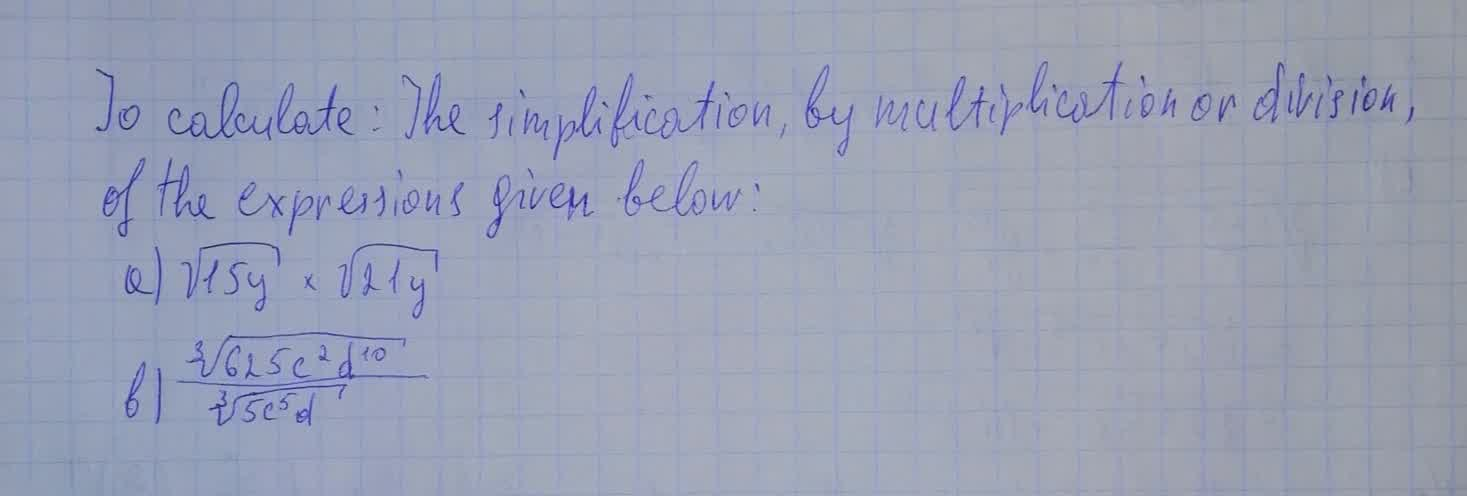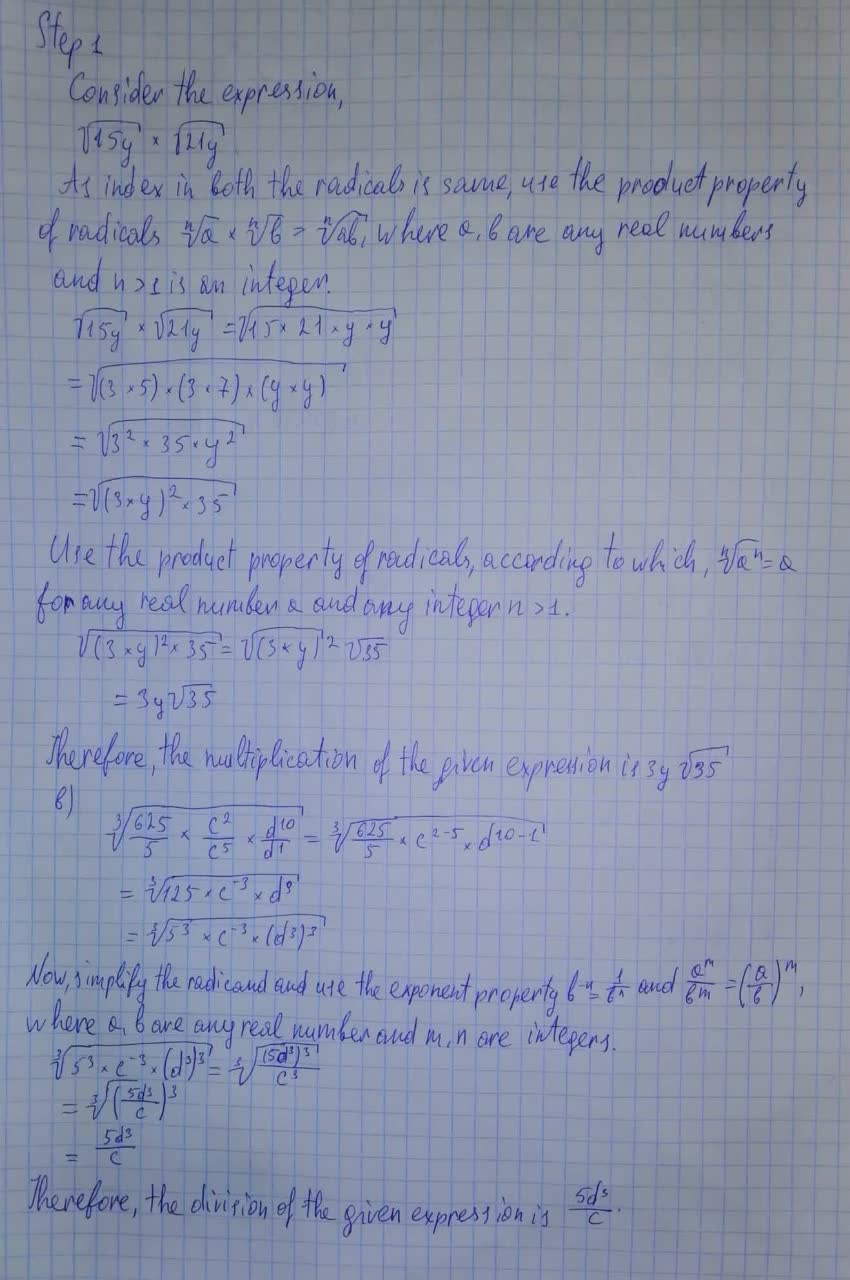# To calculate: The simplification , by multiplication or division , of the expressions given below:a)\sqrt{15y} \cdot \sqrt{21y}sanuluy 2021-08-11 Answered

To calculate: The simplification , by multiplication or division , of the expressions given below:
a) $$\displaystyle\sqrt{{{15}{y}}}\cdot\sqrt{{{21}{y}}}$$
b) $$\frac{\sqrt{625c^2d^{10}}}{\sqrt{5c^5d}}$$• Questions are typically answered in as fast as 30 minutes

### Plainmath recommends

• Get a detailed answer even on the hardest topics.
• Ask an expert for a step-by-step guidance to learn to do it yourself.Fatema Sutton
The answer is given below in the photo